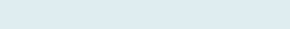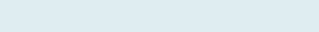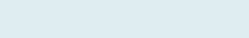# Average only if No Error in Range

After reading this article you will be able to Average numbers if there is an error in the column.

In case you have a column where some cells are filled with numbers and the remaining are #NA (not available). If you want to find average when it met criteria otherwise show an Error.

QUERY

We have two columns Date and Quantity. Our task is to count and if there are at least three integers in a column then give Average otherwise show “Error” as text.

Formula to implement:

=IF(COUNTIF(C3:C5,NA())=0,AVERAGE(C3:C5),”Error”)Count and return average if there is no error in a column otherwise show Error

Functions applicable:

We will be creating a COUNTIIF and IF along with AVERAGE formula in Excel:

• COUNTIF: Returns a count of numbers based on given criteria.
• AVERAGE: Get an average of numbers positioned in one or multiple ranges.
• IF: The function is a handy logical operator that returns a value based on a valid test of criteria, if it matches (TRUE), and another value if it does not.

Formula Explanation (step by step)

Use shortcut F9 function key, tab clicks on evaluating the formula.

Let’s break the formula into multiple steps to understand its application.

First formula

COUNTIF(C3:C5,NA())=0

Syntax: =COUNTIF(range, criteria)

Step 1:It first checks the criteria then counts (C3 to C5) as no number in the given range is matched criteria (NA()=0) so it returns 0.

Second formula:

IF(COUNTIF(C3:C5,NA())=0,AVERAGE(C3:C5),”Error”)

Syntax:  IF(logical_test, value_if_true, [value_if_false])

Step 2:Result: TRUE

As 0=0 so, if there are three consecutive numbers in a column (column C) is TRUE then returns Average otherwise it returns FALSE and shows Error (column D).

Third formula:

AVERAGE(C3:C5)

Syntax: = AVERAGE(number1, [number2], …)

Step 3:Result: 7                ‘Average if three consecutive numbers

It returns Average in the given range (C3 to C5)